# T1 - Essential Fluids - 2023.pptx

Sep. 11, 2023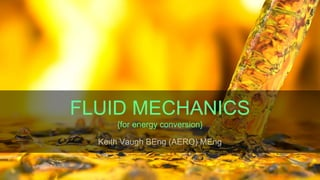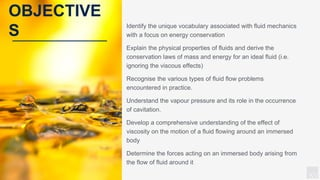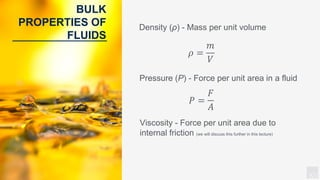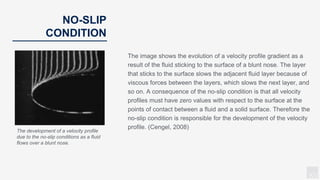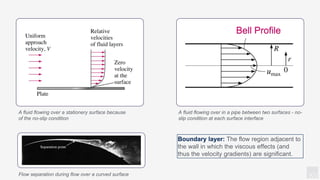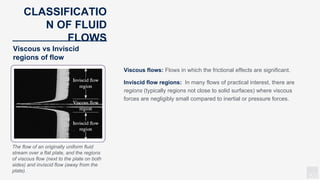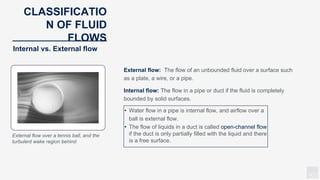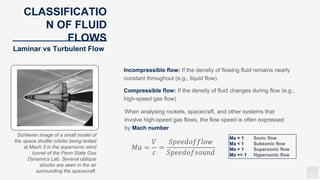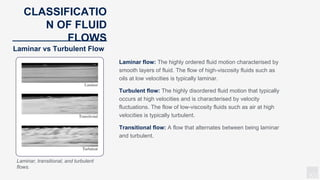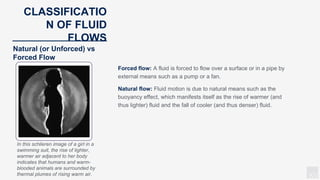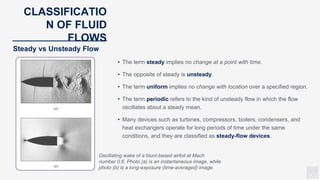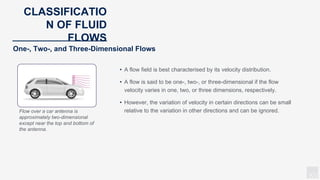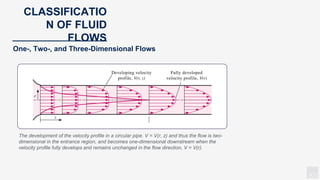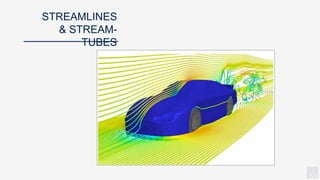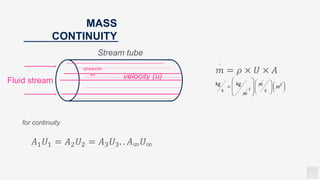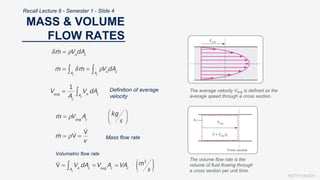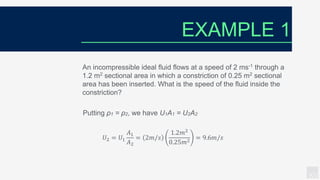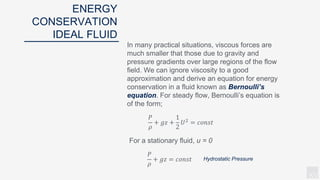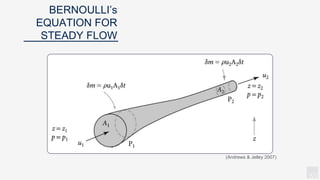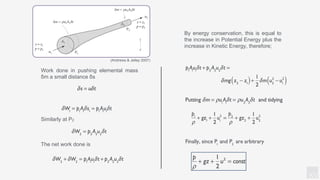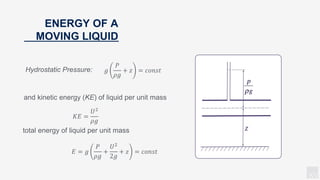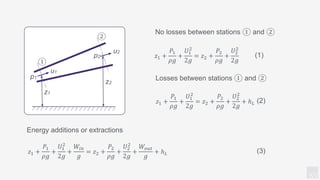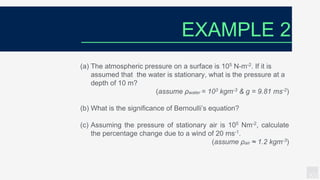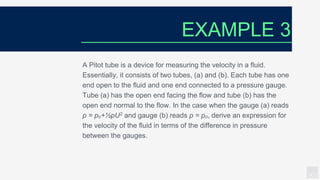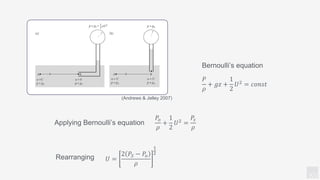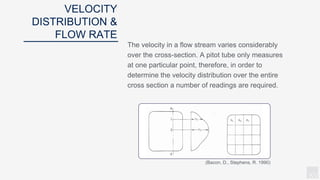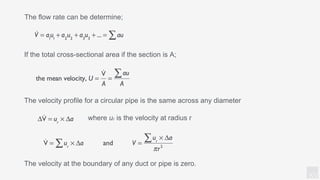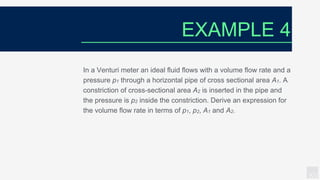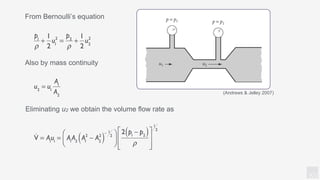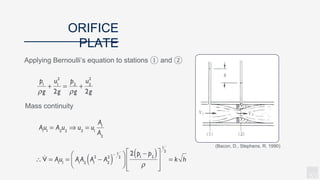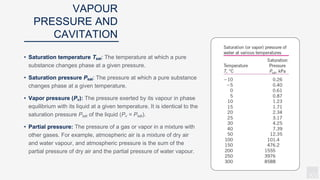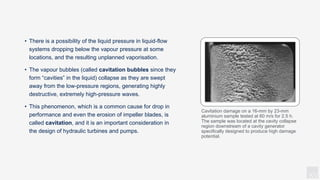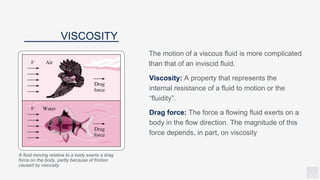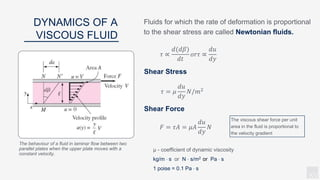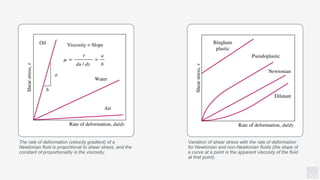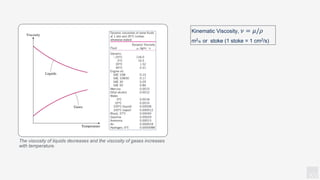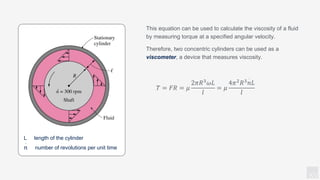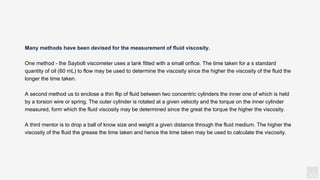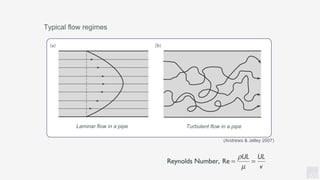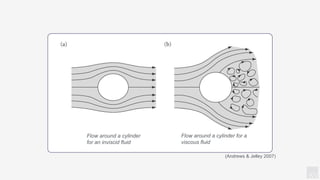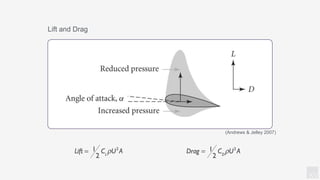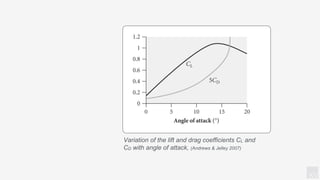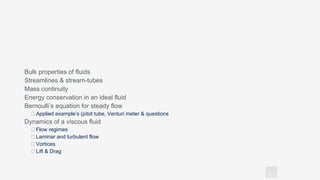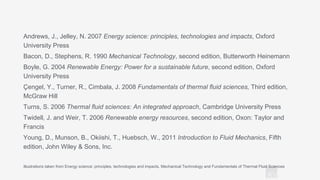1 of 44

### T1 - Essential Fluids - 2023.pptx

• 1. KV FLUID MECHANICS {for energy conversion}
• 2. KV Identify the unique vocabulary associated with fluid mechanics with a focus on energy conservation Explain the physical properties of fluids and derive the conservation laws of mass and energy for an ideal fluid (i.e. ignoring the viscous effects) Recognise the various types of fluid flow problems encountered in practice. Understand the vapour pressure and its role in the occurrence of cavitation. Develop a comprehensive understanding of the effect of viscosity on the motion of a fluid flowing around an immersed body Determine the forces acting on an immersed body arising from the flow of fluid around it OBJECTIVE S
• 3. KV BULK PROPERTIES OF FLUIDS Viscosity - Force per unit area due to internal friction (we will discuss this further in this lecture) Density (ρ) - Mass per unit volume 𝜌 = 𝑚 𝑉 𝑃 = 𝐹 𝐴 Pressure (P) - Force per unit area in a fluid
• 4. KV NO-SLIP CONDITION The image shows the evolution of a velocity profile gradient as a result of the fluid sticking to the surface of a blunt nose. The layer that sticks to the surface slows the adjacent fluid layer because of viscous forces between the layers, which slows the next layer, and so on. A consequence of the no-slip condition is that all velocity profiles must have zero values with respect to the surface at the points of contact between a fluid and a solid surface. Therefore the no-slip condition is responsible for the development of the velocity profile. (Cengel, 2008) The development of a velocity profile due to the no-slip conditions as a fluid flows over a blunt nose.
• 5. KV A fluid flowing over a stationery surface because of the no-slip condition Bell Profile A fluid flowing over in a pipe between two surfaces - no- slip condition at each surface interface Flow separation during flow over a curved surface Boundary layer: The flow region adjacent to the wall in which the viscous effects (and thus the velocity gradients) are significant.
• 6. KV The flow of an originally uniform fluid stream over a flat plate, and the regions of viscous flow (next to the plate on both sides) and inviscid flow (away from the plate). CLASSIFICATIO N OF FLUID FLOWS Viscous vs Inviscid regions of flow Viscous flows: Flows in which the frictional effects are significant. Inviscid flow regions: In many flows of practical interest, there are regions (typically regions not close to solid surfaces) where viscous forces are negligibly small compared to inertial or pressure forces.
• 7. KV External flow over a tennis ball, and the turbulent wake region behind CLASSIFICATIO N OF FLUID FLOWS Internal vs. External flow External flow: The flow of an unbounded fluid over a surface such as a plate, a wire, or a pipe. Internal flow: The flow in a pipe or duct if the fluid is completely bounded by solid surfaces. • Water flow in a pipe is internal flow, and airflow over a ball is external flow. • The flow of liquids in a duct is called open-channel flow if the duct is only partially filled with the liquid and there is a free surface.
• 8. KV Schlieren image of a small model of the space shuttle orbiter being tested at Mach 3 in the supersonic wind tunnel of the Penn State Gas Dynamics Lab. Several oblique shocks are seen in the air surrounding the spacecraft. CLASSIFICATIO N OF FLUID FLOWS Laminar vs Turbulent Flow Incompressible flow: If the density of flowing fluid remains nearly constant throughout (e.g., liquid flow). Compressible flow: If the density of fluid changes during flow (e.g., high-speed gas flow) When analysing rockets, spacecraft, and other systems that involve high-speed gas flows, the flow speed is often expressed by Mach number Ma = 1 Sonic flow Ma < 1 Subsonic flow Ma > 1 Supersonic flow Ma >> 1 Hypersonic flow 𝑀𝑎 = 𝑉 𝑐 = 𝑆𝑝𝑒𝑒𝑑𝑜𝑓𝑓𝑙𝑜𝑤 𝑆𝑝𝑒𝑒𝑑𝑜𝑓𝑠𝑜𝑢𝑛𝑑
• 9. KV CLASSIFICATIO N OF FLUID FLOWS Laminar flow: The highly ordered fluid motion characterised by smooth layers of fluid. The flow of high-viscosity fluids such as oils at low velocities is typically laminar. Turbulent flow: The highly disordered fluid motion that typically occurs at high velocities and is characterised by velocity fluctuations. The flow of low-viscosity fluids such as air at high velocities is typically turbulent. Transitional flow: A flow that alternates between being laminar and turbulent. Laminar vs Turbulent Flow Laminar, transitional, and turbulent flows.
• 10. KV CLASSIFICATIO N OF FLUID FLOWS Forced flow: A fluid is forced to flow over a surface or in a pipe by external means such as a pump or a fan. Natural flow: Fluid motion is due to natural means such as the buoyancy effect, which manifests itself as the rise of warmer (and thus lighter) fluid and the fall of cooler (and thus denser) fluid. Natural (or Unforced) vs Forced Flow In this schlieren image of a girl in a swimming suit, the rise of lighter, warmer air adjacent to her body indicates that humans and warm- blooded animals are surrounded by thermal plumes of rising warm air.
• 11. KV CLASSIFICATIO N OF FLUID FLOWS • The term steady implies no change at a point with time. • The opposite of steady is unsteady. • The term uniform implies no change with location over a specified region. • The term periodic refers to the kind of unsteady flow in which the flow oscillates about a steady mean. • Many devices such as turbines, compressors, boilers, condensers, and heat exchangers operate for long periods of time under the same conditions, and they are classified as steady-flow devices. Steady vs Unsteady Flow Oscillating wake of a blunt-based airfoil at Mach number 0.6. Photo (a) is an instantaneous image, while photo (b) is a long-exposure (time-averaged) image.
• 12. KV CLASSIFICATIO N OF FLUID FLOWS • A flow field is best characterised by its velocity distribution. • A flow is said to be one-, two-, or three-dimensional if the flow velocity varies in one, two, or three dimensions, respectively. • However, the variation of velocity in certain directions can be small relative to the variation in other directions and can be ignored. One-, Two-, and Three-Dimensional Flows Flow over a car antenna is approximately two-dimensional except near the top and bottom of the antenna.
• 13. KV CLASSIFICATIO N OF FLUID FLOWS One-, Two-, and Three-Dimensional Flows The development of the velocity profile in a circular pipe. V = V(r, z) and thus the flow is two- dimensional in the entrance region, and becomes one-dimensional downstream when the velocity profile fully develops and remains unchanged in the flow direction, V = V(r).
• 14. KV STREAMLINES & STREAM- TUBES
• 15. KV Fluid stream velocity (u) Stream tube streamlin es MASS CONTINUITY 𝑚 · = 𝜌 × 𝑈 × 𝐴 for continuity 𝐴1𝑈1 = 𝐴2𝑈2 = 𝐴3𝑈3. . 𝐴∞𝑈∞
• 16. KEITH VAUGH MASS & VOLUME FLOW RATES Mass flow rate The average velocity Vavg is defined as the average speed through a cross section. Definition of average velocity Volumetric flow rate The volume flow rate is the volume of fluid flowing through a cross section per unit time. Recall Lecture 8 - Semester 1 - Slide 4
• 17. KV An incompressible ideal fluid flows at a speed of 2 ms-1 through a 1.2 m2 sectional area in which a constriction of 0.25 m2 sectional area has been inserted. What is the speed of the fluid inside the constriction? Putting ρ1 = ρ2, we have U1A1 = U2A2 EXAMPLE 1 𝑈2 = 𝑈1 𝐴1 𝐴2 = 2𝑚/𝑠 1.2𝑚2 0.25𝑚2 = 9.6𝑚/𝑠
• 18. KV In many practical situations, viscous forces are much smaller that those due to gravity and pressure gradients over large regions of the flow field. We can ignore viscosity to a good approximation and derive an equation for energy conservation in a fluid known as Bernoulli’s equation. For steady flow, Bernoulli’s equation is of the form; For a stationary fluid, u = 0 ENERGY CONSERVATION IDEAL FLUID 𝑃 𝜌 + 𝑔𝑧 + 1 2 𝑈2 = 𝑐𝑜𝑛𝑠𝑡 𝑃 𝜌 + 𝑔𝑧 = 𝑐𝑜𝑛𝑠𝑡 Hydrostatic Pressure
• 19. KV BERNOULLI’s EQUATION FOR STEADY FLOW (Andrews & Jelley 2007)
• 20. KV Work done in pushing elemental mass δm a small distance δs Similarly at P2 The net work done is By energy conservation, this is equal to the increase in Potential Energy plus the increase in Kinetic Energy, therefore; (Andrews & Jelley 2007)
• 21. KV and kinetic energy (KE) of liquid per unit mass total energy of liquid per unit mass z ENERGY OF A MOVING LIQUID 𝑔 𝑃 𝜌𝑔 + 𝑧 = 𝑐𝑜𝑛𝑠𝑡 Hydrostatic Pressure: 𝐸 = 𝑔 𝑃 𝜌𝑔 + 𝑈2 2𝑔 + 𝑧 = 𝑐𝑜𝑛𝑠𝑡 𝐾𝐸 = 𝑈2 𝜌𝑔
• 22. KV No losses between stations ① and ② Losses between stations ① and ② Energy additions or extractions (2) ① ② z1 z2 p1 p2 u1 u2 𝑧1 + 𝑃1 𝜌𝑔 + 𝑈1 2 2𝑔 = 𝑧2 + 𝑃2 𝜌𝑔 + 𝑈2 2 2𝑔 𝑧1 + 𝑃1 𝜌𝑔 + 𝑈1 2 2𝑔 = 𝑧2 + 𝑃2 𝜌𝑔 + 𝑈2 2 2𝑔 + ℎ𝐿 𝑧1 + 𝑃1 𝜌𝑔 + 𝑈1 2 2𝑔 + 𝑊𝑖𝑛 𝑔 = 𝑧2 + 𝑃2 𝜌𝑔 + 𝑈2 2 2𝑔 + 𝑊𝑜𝑢𝑡 𝑔 + ℎ𝐿 (3) (1)
• 23. KV (a) The atmospheric pressure on a surface is 105 N-m-2. If it is assumed that the water is stationary, what is the pressure at a depth of 10 m? (assume ρwater = 103 kgm-3 & g = 9.81 ms-2) (b) What is the significance of Bernoulli’s equation? (c) Assuming the pressure of stationary air is 105 Nm-2, calculate the percentage change due to a wind of 20 ms-1. (assume ρair ≈ 1.2 kgm-3) EXAMPLE 2
• 24. KV A Pitot tube is a device for measuring the velocity in a fluid. Essentially, it consists of two tubes, (a) and (b). Each tube has one end open to the fluid and one end connected to a pressure gauge. Tube (a) has the open end facing the flow and tube (b) has the open end normal to the flow. In the case when the gauge (a) reads p = po+½pU2 and gauge (b) reads p = po, derive an expression for the velocity of the fluid in terms of the difference in pressure between the gauges. EXAMPLE 3
• 25. KV Applying Bernoulli’s equation Bernoulli’s equation Rearranging (Andrews & Jelley 2007) 𝑃 𝜌 + 𝑔𝑧 + 1 2 𝑈2 = 𝑐𝑜𝑛𝑠𝑡 𝑃𝑜 𝜌 + 1 2 𝑈2 = 𝑃𝑠 𝜌 𝑈 = 2 𝑃𝑆 − 𝑃𝑜 𝜌 1 2
• 26. KV The velocity in a flow stream varies considerably over the cross-section. A pitot tube only measures at one particular point, therefore, in order to determine the velocity distribution over the entire cross section a number of readings are required. VELOCITY DISTRIBUTION & FLOW RATE (Bacon, D., Stephens, R. 1990)
• 27. KV The flow rate can be determine; If the total cross-sectional area if the section is A; The velocity profile for a circular pipe is the same across any diameter where ur is the velocity at radius r The velocity at the boundary of any duct or pipe is zero.
• 28. KV In a Venturi meter an ideal fluid flows with a volume flow rate and a pressure p1 through a horizontal pipe of cross sectional area A1. A constriction of cross-sectional area A2 is inserted in the pipe and the pressure is p2 inside the constriction. Derive an expression for the volume flow rate in terms of p1, p2, A1 and A2. EXAMPLE 4
• 29. KV From Bernoulli’s equation Also by mass continuity Eliminating u2 we obtain the volume flow rate as (Andrews & Jelley 2007)
• 30. KV Applying Bernoulli’s equation to stations ① and ② Mass continuity ORIFICE PLATE (Bacon, D., Stephens, R. 1990)
• 31. KV • Saturation temperature Tsat: The temperature at which a pure substance changes phase at a given pressure. • Saturation pressure Psat: The pressure at which a pure substance changes phase at a given temperature. • Vapor pressure (Pv): The pressure exerted by its vapour in phase equilibrium with its liquid at a given temperature. It is identical to the saturation pressure Psat of the liquid (Pv = Psat). • Partial pressure: The pressure of a gas or vapor in a mixture with other gases. For example, atmospheric air is a mixture of dry air and water vapour, and atmospheric pressure is the sum of the partial pressure of dry air and the partial pressure of water vapour. VAPOUR PRESSURE AND CAVITATION
• 32. KV • There is a possibility of the liquid pressure in liquid-flow systems dropping below the vapour pressure at some locations, and the resulting unplanned vaporisation. • The vapour bubbles (called cavitation bubbles since they form “cavities” in the liquid) collapse as they are swept away from the low-pressure regions, generating highly destructive, extremely high-pressure waves. • This phenomenon, which is a common cause for drop in performance and even the erosion of impeller blades, is called cavitation, and it is an important consideration in the design of hydraulic turbines and pumps. Cavitation damage on a 16-mm by 23-mm aluminium sample tested at 60 m/s for 2.5 h. The sample was located at the cavity collapse region downstream of a cavity generator specifically designed to produce high damage potential.
• 33. KV Viscosity: A property that represents the internal resistance of a fluid to motion or the “fluidity”. Drag force: The force a flowing fluid exerts on a body in the flow direction. The magnitude of this force depends, in part, on viscosity VISCOSITY A fluid moving relative to a body exerts a drag force on the body, partly because of friction caused by viscosity. The motion of a viscous fluid is more complicated than that of an inviscid fluid.
• 34. KV Fluids for which the rate of deformation is proportional to the shear stress are called Newtonian fluids. DYNAMICS OF A VISCOUS FLUID The viscous shear force per unit area in the fluid is proportional to the velocity gradient μ - coefficient of dynamic viscosity kg/m  s or N  s/m2 or Pa  s 1 poise = 0.1 Pa  s The behaviour of a fluid in laminar flow between two parallel plates when the upper plate moves with a constant velocity. 𝜏 ∝ 𝑑(𝑑𝛽) 𝑑𝑡 𝑜𝑟𝜏 ∝ 𝑑𝑢 𝑑𝑦 𝜏 = 𝜇 𝑑𝑢 𝑑𝑦 𝑁/𝑚2 𝐹 = 𝜏𝐴 = 𝜇𝐴 𝑑𝑢 𝑑𝑦 𝑁 Shear Stress Shear Force
• 35. KV The rate of deformation (velocity gradient) of a Newtonian fluid is proportional to shear stress, and the constant of proportionality is the viscosity. Variation of shear stress with the rate of deformation for Newtonian and non-Newtonian fluids (the slope of a curve at a point is the apparent viscosity of the fluid at that point).
• 36. KV The viscosity of liquids decreases and the viscosity of gases increases with temperature. Kinematic Viscosity, 𝜈 = 𝜇/𝜌 m2/s or stoke (1 stoke = 1 cm2/s)
• 37. KV L length of the cylinder 𝑛 · number of revolutions per unit time This equation can be used to calculate the viscosity of a fluid by measuring torque at a specified angular velocity. Therefore, two concentric cylinders can be used as a viscometer, a device that measures viscosity. 𝑇 = 𝐹𝑅 = 𝜇 2𝜋𝑅3 𝜔𝐿 𝑙 = 𝜇 4𝜋2 𝑅3 𝑛 · 𝐿 𝑙
• 38. KV Many methods have been devised for the measurement of fluid viscosity. One method - the Saybolt viscometer uses a tank fitted with a small orifice. The time taken for a s standard quantity of oil (60 mL) to flow may be used to determine the viscosity since the higher the viscosity of the fluid the longer the time taken. A second method us to enclose a thin flip of fluid between two concentric cylinders the inner one of which is held by a torsion wire or spring. The outer cylinder is rotated at a given velocity and the torque on the inner cylinder measured, form which the fluid viscosity may be determined since the great the torque the higher the viscosity. A third mentor is to drop a ball of know size and weight a given distance through the fluid medium. The higher the viscosity of the fluid the grease the time taken and hence the time taken may be used to calculate the viscosity.
• 39. KV Laminar flow in a pipe Turbulent flow in a pipe Typical flow regimes (Andrews & Jelley 2007)
• 40. KV Flow around a cylinder for an inviscid fluid Flow around a cylinder for a viscous fluid (Andrews & Jelley 2007)
• 41. KV Lift and Drag (Andrews & Jelley 2007)
• 42. KV Variation of the lift and drag coefficients CL and CD with angle of attack, (Andrews & Jelley 2007)
• 43. KV Bulk properties of fluids Streamlines & stream-tubes Mass continuity Energy conservation in an ideal fluid Bernoulli’s equation for steady flow Applied example’s (pitot tube, Venturi meter & questions Dynamics of a viscous fluid Flow regimes Laminar and turbulent flow Vortices Lift & Drag
• 44. KV Andrews, J., Jelley, N. 2007 Energy science: principles, technologies and impacts, Oxford University Press Bacon, D., Stephens, R. 1990 Mechanical Technology, second edition, Butterworth Heinemann Boyle, G. 2004 Renewable Energy: Power for a sustainable future, second edition, Oxford University Press Çengel, Y., Turner, R., Cimbala, J. 2008 Fundamentals of thermal fluid sciences, Third edition, McGraw Hill Turns, S. 2006 Thermal fluid sciences: An integrated approach, Cambridge University Press Twidell, J. and Weir, T. 2006 Renewable energy resources, second edition, Oxon: Taylor and Francis Young, D., Munson, B., Okiishi, T., Huebsch, W., 2011 Introduction to Fluid Mechanics, Fifth edition, John Wiley & Sons, Inc. Illustrations taken from Energy science: principles, technologies and impacts, Mechanical Technology and Fundamentals of Thermal Fluid Sciences

### Editor's Notes

1. To appreciate energy conversion such as hydro, wave, tidal and wind power a detailed knowledge of fluid mechanics is essential. During the course of this lecture, a brief summary of the basic physical properties of fluids is provided and the conservation laws of mass and energy for an ideal (or inviscid) fluid are derived. The application of the conservation laws to situations of practical interest are also explored to illustrate how useful information about the flow can be derived. Finally, the effect of viscosity on the motion of a fluid around an immersed body (such as a turbine blade) and how the flow determines the forces acting on the body of interest.
2. Density - Mass per unit volume of a fluid. For the purposes of this module, Density is assumed to be constant i.e. that the flow of the fluid is incompressible (small variations in pressure arising from fluid motion in comparison to atmospheric pressure). Pressure - Force per unit area, and acts in the normal direction to the surface of a body in a fluid. Its units are the pascal or N-m^2 Viscosity - Force per unit area due to internal friction in a fluid arising from the relative motion between neighbouring elements in a fluid. Viscous forces act in the tangential direction to the surface of a body immersed in a flow. (consider a deck of cards.
3. Density - Mass per unit volume of a fluid. For the purposes of this module, Density is assumed to be constant i.e. that the flow of the fluid is incompressible (small variations in pressure arising from fluid motion in comparison to atmospheric pressure). Pressure - Force per unit area, and acts in the normal direction to the surface of a body in a fluid. Its units are the pascal or N-m^2 Viscosity - Force per unit area due to internal friction in a fluid arising from the relative motion between neighbouring elements in a fluid. Viscous forces act in the tangential direction to the surface of a body immersed in a flow. (consider a deck of cards.
4. Density - Mass per unit volume of a fluid. For the purposes of this module, Density is assumed to be constant i.e. that the flow of the fluid is incompressible (small variations in pressure arising from fluid motion in comparison to atmospheric pressure). Pressure - Force per unit area, and acts in the normal direction to the surface of a body in a fluid. Its units are the pascal or N-m^2 Viscosity - Force per unit area due to internal friction in a fluid arising from the relative motion between neighbouring elements in a fluid. Viscous forces act in the tangential direction to the surface of a body immersed in a flow. (consider a deck of cards.
5. Density - Mass per unit volume of a fluid. For the purposes of this module, Density is assumed to be constant i.e. that the flow of the fluid is incompressible (small variations in pressure arising from fluid motion in comparison to atmospheric pressure). Pressure - Force per unit area, and acts in the normal direction to the surface of a body in a fluid. Its units are the pascal or N-m^2 Viscosity - Force per unit area due to internal friction in a fluid arising from the relative motion between neighbouring elements in a fluid. Viscous forces act in the tangential direction to the surface of a body immersed in a flow. (consider a deck of cards.
6. Density - Mass per unit volume of a fluid. For the purposes of this module, Density is assumed to be constant i.e. that the flow of the fluid is incompressible (small variations in pressure arising from fluid motion in comparison to atmospheric pressure). Pressure - Force per unit area, and acts in the normal direction to the surface of a body in a fluid. Its units are the pascal or N-m^2 Viscosity - Force per unit area due to internal friction in a fluid arising from the relative motion between neighbouring elements in a fluid. Viscous forces act in the tangential direction to the surface of a body immersed in a flow. (consider a deck of cards.
7. Density - Mass per unit volume of a fluid. For the purposes of this module, Density is assumed to be constant i.e. that the flow of the fluid is incompressible (small variations in pressure arising from fluid motion in comparison to atmospheric pressure). Pressure - Force per unit area, and acts in the normal direction to the surface of a body in a fluid. Its units are the pascal or N-m^2 Viscosity - Force per unit area due to internal friction in a fluid arising from the relative motion between neighbouring elements in a fluid. Viscous forces act in the tangential direction to the surface of a body immersed in a flow. (consider a deck of cards.
8. Density - Mass per unit volume of a fluid. For the purposes of this module, Density is assumed to be constant i.e. that the flow of the fluid is incompressible (small variations in pressure arising from fluid motion in comparison to atmospheric pressure). Pressure - Force per unit area, and acts in the normal direction to the surface of a body in a fluid. Its units are the pascal or N-m^2 Viscosity - Force per unit area due to internal friction in a fluid arising from the relative motion between neighbouring elements in a fluid. Viscous forces act in the tangential direction to the surface of a body immersed in a flow. (consider a deck of cards.
9. Density - Mass per unit volume of a fluid. For the purposes of this module, Density is assumed to be constant i.e. that the flow of the fluid is incompressible (small variations in pressure arising from fluid motion in comparison to atmospheric pressure). Pressure - Force per unit area, and acts in the normal direction to the surface of a body in a fluid. Its units are the pascal or N-m^2 Viscosity - Force per unit area due to internal friction in a fluid arising from the relative motion between neighbouring elements in a fluid. Viscous forces act in the tangential direction to the surface of a body immersed in a flow. (consider a deck of cards.
10. Density - Mass per unit volume of a fluid. For the purposes of this module, Density is assumed to be constant i.e. that the flow of the fluid is incompressible (small variations in pressure arising from fluid motion in comparison to atmospheric pressure). Pressure - Force per unit area, and acts in the normal direction to the surface of a body in a fluid. Its units are the pascal or N-m^2 Viscosity - Force per unit area due to internal friction in a fluid arising from the relative motion between neighbouring elements in a fluid. Viscous forces act in the tangential direction to the surface of a body immersed in a flow. (consider a deck of cards.
11. Density - Mass per unit volume of a fluid. For the purposes of this module, Density is assumed to be constant i.e. that the flow of the fluid is incompressible (small variations in pressure arising from fluid motion in comparison to atmospheric pressure). Pressure - Force per unit area, and acts in the normal direction to the surface of a body in a fluid. Its units are the pascal or N-m^2 Viscosity - Force per unit area due to internal friction in a fluid arising from the relative motion between neighbouring elements in a fluid. Viscous forces act in the tangential direction to the surface of a body immersed in a flow. (consider a deck of cards.
12. Density - Mass per unit volume of a fluid. For the purposes of this module, Density is assumed to be constant i.e. that the flow of the fluid is incompressible (small variations in pressure arising from fluid motion in comparison to atmospheric pressure). Pressure - Force per unit area, and acts in the normal direction to the surface of a body in a fluid. Its units are the pascal or N-m^2 Viscosity - Force per unit area due to internal friction in a fluid arising from the relative motion between neighbouring elements in a fluid. Viscous forces act in the tangential direction to the surface of a body immersed in a flow. (consider a deck of cards.
13. A useful concept to visualize a velocity field is to imagine a set of streamlines para;;e; to the direction of motion at all points in the fluid. Any element of mass in the fluid flows along a notational stream-tube bounded by neighbouring streamlines. In practice, streamlines can be visualised by injecting small particles into the fluid e.g. smoke can be used in wind tunnels to visualise fluid flow around objects.
14. Also known as conservation of mass. Consider flow along a stream tube in a steady velocity field. The direction of flow is parallel to the boundries and at any point within the stream tube, the speed of the fluid (u) and the cross-sectional area (A). Therefore the fluid is confined to the stream tube and the mass flow per second is constant. Therefore: Density * Velocity * Cross-sectional Area = Constant Therefore the speed of fluid is inversely proportional to the cross-sectional area of the stream tube.
15. note the use of symbols here. DON’T confuse Volume (V) and velocity (V)
16. For a stationary fluid, u = 0 everywhere in the fluid therefore this equation reduces to which is the equation for hydrostatic pressure. It shows that the fluid at a given depth is all the same at the same pressure.
17. Consider the steady flow of an ideal fluid in the control volume shown. The height (z), cross sectional area (A), speed (u) and pressure are denoted. The increase in gravitational potential energy of a mass δm of fluid between z1 and z2 is δmg(z2-z1) In a short time interval (δt) the mass of fluid entering the control volume at P1 is δm=ρu1A1δt and the mass exiting at P2 is δm=ρu2A2δt
18. In order for the fluid to enter the control volume it has to do work to overcome the pressure p1 exerted by the fluid. The work done in pushing the elemental mass δm a small distance δs = uδt at P1 is δW1= Pressure * Area * Distance moved = Pressure * Area * Velocity * Change in time. Remember F = P*A Similarly, the work done in pushing the elemental mass out of the control volume at P2 is δW2=-p2A2u2δt. The net work done is δW1+δW2
19. Consider a liquid at a pressure p, moving with a velocity (u) at a height Z above datum level If a tube were inserted in the top of the pipe, the liquid would rise up the tube a distance of p/ρg and this is equivalent to an additional height of liquid relative to datum level. Etotal equation represents the specific energy of the liquid. Each of the quantities in the brackets have the units of length and are termed heads, i.e Z is referred to as the potential head of the liquid, p/ρg as the pressure head and V2/2g as the velocity head.
20. Consider stations ① and ② of the inclined pipe illustrated. If there are no losses between these two sections, and no energy changes resulting from heat transfer of work, then the total energy will remain constant, therefore stations ① and ② can be set equal to one another, equ (1) If however losses do occur between the two stations, then equation equ (2) When energy is added to the fluid by a device such as a pump, or when energy is extracted by a turbine, these need also be accounted for equ (3). w is the specific work in J/kg NOTE - V1 and V2 indicated in diagram are u1 and u2 in derivation
21. Consider the fluid flowing along the stream-line AB. In case (a), the fluid slows down as it approaches the stagnation point B. Putting u = U, p = po at A and u = 0, p = ps at B and applying bernoulli’s equation we get In example (and the previous question), ps is measured by tube (a) and p0 measured by tube (b). NOTE - ps is larger than p0 by an amount ps - p0 = ρU2. The quantities ρU2, p0 and ps = p0 + ρU2 are called the dynamic pressure, static pressure and total pressure respectively.
22. The velocity profile is obtained by traversing the pitot tube along the line AA. The cross-section is divided into convenient areas, a1, a2, a3, etc... and the velocity at the centre of each area is determined
23. A venturi meter is a device which is used to measure the rate of flow through a pipe. NOTE - V1 and V2 indicated in diagram are u1 and u2 in derivation
24. An Orifice plate is another means of determining the fluid flow in a pipe and works on the same principle of the Venturi meter. The position of the Vena contract can be difficult establish and therefore the area A2. The constant k is determined experimentally when it will incorporate the coefficient of discharge. If h is small so that ρ is approximately constant then the this equation can be used for compressible fluid flow. NOTE - V1 and V2 indicated in diagram are u1 and u2 in derivation
25. Let us consider a simple case of laminar flow between two parallel plates separated by a small distance d. The upper plate moves at a constant velocity U while the lower plate remains at rest. At the plate fluid interface in both cases there is no velocity due to the strong forces of attraction. Therefore the velocity profile in the fluid is given by
26. Let us consider a simple case of laminar flow between two parallel plates separated by a small distance d. The upper plate moves at a constant velocity U while the lower plate remains at rest. At the plate fluid interface in both cases there is no velocity due to the strong forces of attraction. Therefore the velocity profile in the fluid is given by
27. Let us consider a simple case of laminar flow between two parallel plates separated by a small distance d. The upper plate moves at a constant velocity U while the lower plate remains at rest. At the plate fluid interface in both cases there is no velocity due to the strong forces of attraction. Therefore the velocity profile in the fluid is given by
28. Let us consider a simple case of laminar flow between two parallel plates separated by a small distance d. The upper plate moves at a constant velocity U while the lower plate remains at rest. At the plate fluid interface in both cases there is no velocity due to the strong forces of attraction. Therefore the velocity profile in the fluid is given by
29. Let us consider a simple case of laminar flow between two parallel plates separated by a small distance d. The upper plate moves at a constant velocity U while the lower plate remains at rest. At the plate fluid interface in both cases there is no velocity due to the strong forces of attraction. Therefore the velocity profile in the fluid is given by
30. Let us consider a simple case of laminar flow between two parallel plates separated by a small distance d. The upper plate moves at a constant velocity U while the lower plate remains at rest. At the plate fluid interface in both cases there is no velocity due to the strong forces of attraction. Therefore the velocity profile in the fluid is given by
31. Let us consider a simple case of laminar flow between two parallel plates separated by a small distance d. The upper plate moves at a constant velocity U while the lower plate remains at rest. At the plate fluid interface in both cases there is no velocity due to the strong forces of attraction. Therefore the velocity profile in the fluid is given by
32. Let us consider a simple case of laminar flow between two parallel plates separated by a small distance d. The upper plate moves at a constant velocity U while the lower plate remains at rest. At the plate fluid interface in both cases there is no velocity due to the strong forces of attraction. Therefore the velocity profile in the fluid is given by
33. A viscous fluid can exhibit two different kinds of flow regimes, Laminar and Turbulent In laminar flow, the fluid slides along distinct stream-tubes and tends to be quite stable, Turbulent flow is disorderly and unstable The flow that exists in an y given situation depends on the ratio of the inertial force to the viscous force. The magnitude of this ratio is given by Reynolds number, where U is the velocity, L is the length and v = μ/ρ is the kinematic viscosity. Reynolds observed that flows at small Re where laminar while flow at high Re contained regions of turbulence
34. For the inviscid flow the velocity fields in the upstream and downstream regions are symmetrical, therefore the corresponding pressure distribution is symmetrical. It follows that the net force exerted by the fluid on the cylinder is zero. This is an example of d’Alembert’s paradox For a body immersed in a viscious fluid, the component of velocity tangential to the surface of the fluid is zero at all points. At large Re numbers (Re>>1), the viscous force is negligible in the bulk of the fluid but us very significant in a viscous boundary layer close to the surface of the body. Rotational components of flow know as vorticity are generated within the boundary layer. As a certain point, the seperation point, the boundary layer becomes detached from the surface and vorticity are discharged into the body of the fluid. The vorticity are transported downstream side of the cylinder in the wake. Therefore the pressure distributions on the upstream and downstream sides of the cylinder are not symmetrical in the case of a viscous fluid. As a result, the cylinder experiences a net force in the direction of motion known as drag. In the case of a spinning cylinder, a force called life arises at right angles to the direction of flow.
35. CL and CD are dimensionless constants know as the coefficient of Lift and the coefficient of Drag respectively. Lift and drag can be changed by altering the shape of a wing i.e. ailerons. For small angles of attack, the pressure distribution ont he upper surface of an aerofoil is significantly lower than that on the lower surface which results is in a net lift force.
36. CL and CD are dimensionless constants know as the coefficient of Lift and the coefficient of Drag respectively. Lift and drag can be changed by altering the shape of a wing i.e. ailerons. For small angles of attack, the pressure distribution on the upper surface of an aerofoil is significantly lower than that on the lower surface which results is in a net lift force.